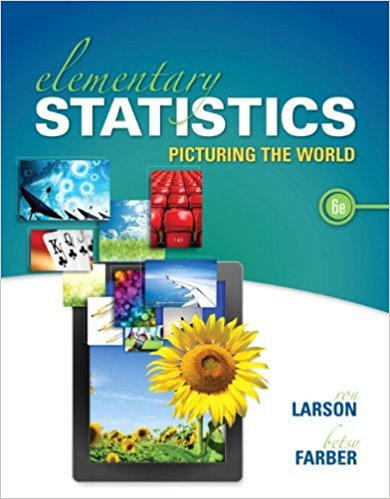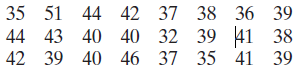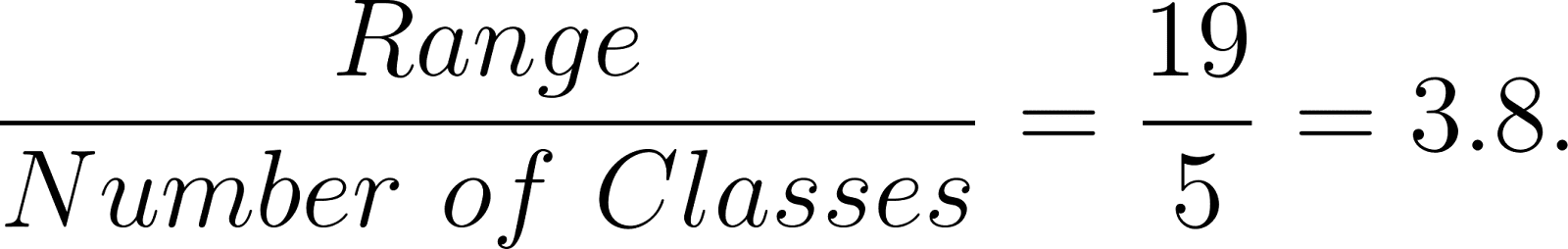×
Get Full Access to Elementary Statistics: Picturing The World - 6 Edition - Chapter 2.1 - Problem 32e
Get Full Access to Elementary Statistics: Picturing The World - 6 Edition - Chapter 2.1 - Problem 32e

×

# Answer: Constructing a Frequency Distribution and aISBN: 9780321911216 66

## Solution for problem 32E Chapter 2.1

Elementary Statistics: Picturing the World | 6th Edition

• Textbook Solutions
• 2901 Step-by-step solutions solved by professors and subject experts
• Get 24/7 help from StudySoup virtual teaching assistantsElementary Statistics: Picturing the World | 6th Edition

4 5 1 278 Reviews
27
3
Problem 32E

Constructing a Frequency Distribution and a Frequency Histogram

In Exercises 31–34, construct a frequency distribution and a frequency histogram for the data set using the indicated number of classes. Describe any patterns.

Pepper Pungencies

Number of classes: 5

Data set: Pungencies (in thousands of Scoville units) of 24 tabasco peppersStep-by-Step Solution:

Problem 32E

Constructing a Frequency Distribution and a Frequency Histogram In Exercise, construct a frequency distribution and a frequency histogram for the data set using the indicated number of classes. Describe any patterns.

Pepper Pungencies

Number of classes: 5

Data set: Pungencies (in thousands of Scoville units) of 24 tabasco peppers

 35 51 44 42 37 38 36 39 44 43 40 40 32 39 41 38 42 39 40 46 37 35 41 39

Step by Step Solution

Step 1 of 3

The number of classes stated in the problem = 5

The minimum data entry is 32 and the maximum data entry is 51, so the range is

Range = 51 - 32 = 19.

Divide the range by the number of classes and round up to find the class width, ie;Class width =

Round up to the next convenient number, 4.

The minimum data entry is 32 and add the class width of 4 to the lower limit of each previous class to get the lower limits of the remaining four classes.

We have the upper limit of the first class as 35. Then the first class is 32 - 35. The upper limit of the first class, 35 is one less than the lower limit of the second class. Then add the class width of 4 to the upper limit of each previous class to get the upper limits of the remaining four classes. In this way we can write each class, as shown below,

 Lower limit Upper limit 32 35 36 39 40 43 44 47 48 51

So, we have the lower and upper limit of each class. Now we can construct a frequency distribution.

A frequency distribution is a table that shows classes or intervals of data entries with a count of the number of entries in each class. The frequency f of a class is the number of data entries in the class. The frequency distribution of the above data set is shown below,

 Class Frequency, f 32-35 3 36-39 9 40-43 8 44-47 3 48-51 1

Step 2 of 3

Step 3 of 3

##### ISBN: 9780321911216

Since the solution to 32E from 2.1 chapter was answered, more than 922 students have viewed the full step-by-step answer. This full solution covers the following key subjects: frequency, histogram, pungencies, set, data. This expansive textbook survival guide covers 66 chapters, and 3049 solutions. This textbook survival guide was created for the textbook: Elementary Statistics: Picturing the World , edition: 6. The answer to “?Constructing a Frequency Distribution and a Frequency HistogramIn Exercises 31–34, construct a frequency distribution and a frequency histogram for the data set using the indicated number of classes. Describe any patterns.Pepper PungenciesNumber of classes: 5Data set: Pungencies (in thousands of Scoville units) of 24 tabasco peppers” is broken down into a number of easy to follow steps, and 46 words. The full step-by-step solution to problem: 32E from chapter: 2.1 was answered by , our top Statistics solution expert on 08/25/17, 09:43AM. Elementary Statistics: Picturing the World was written by and is associated to the ISBN: 9780321911216.

Unlock Textbook Solution